# Problem of the Month (December 2005)

This month we consider several problems concerning finite sets of points in the plane.

Problem #1. Consider n marksmen standing in a field (so that all their pairwise distances are different). Each round, each marksman simultaneously shoots and kills the closest marksman. This is repeated until fewer than 2 marksmen remain alive. How do we arrange n marksmen so that the maximum number of rounds are necessary? (We count a surviving marksman as an additional half round to prefer it over the same number of rounds in which everyone is killed.)

A recent Macalester College problem of the week hints at an easier question: for n marksmen, what is the smallest number of marksmen that can be killed during the first round of shooting?

Problem #2. The Erdös distance problem is well-known. Given n points in the plane, what is the fewest number of different distances Δ(n) they can determine? For large n, Δ(n) is known to be of the order n/√(log n). But what are the small values of Δ(n)?

Problem #3. A set of n points in the plane is called k-hidden if each point can "not see" exactly k other points because they are hidden behind other points. For a given k, what is the smallest number of points that can be k-hidden? For a given k, which n can exhibit a k-hidden arrangement?

Problem #4. A matchstick graph is a planar graph whose edges are unit line segments. The problem of finding the smallest regular matchstick graphs (finding arrangements of non-crossing matchsticks so that exactly n matchsticks meet at every matchstick end) is well-known. The smallest known n-regular matchstick graphs (for 2≤n≤4) are shown below (the last graph is due to H. Harborth and M. Timm). No 5-regular matchstick graph exists.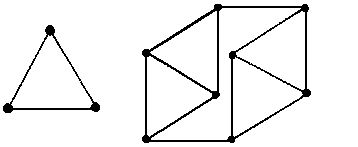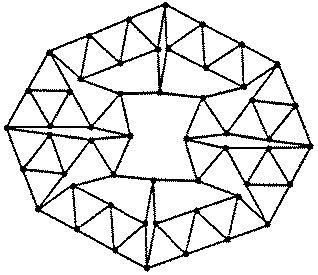We search for the smallest matchstick graphs whose vertices have 2 different degrees. An m/n matchstick graph is a matchstick graph where the vertices have degree m or n, with each occurring at least once. An equal m/n matchstick graph is a matchstick graph where half the vertices have degree m and half have degree n. Find the smallest possible m/n matchstick graphs and equal m/n matchstick graphs for small m and n.

Problem #1:

Joe DeVincentis noted that for n≤8, the best arrangement achieves r(n)=n/2 rounds, since only 2 marksmen die per round.

In 2018, Alexander Ravsky noted it is clear that r(n) is non-decreasing and r(n+2) ≤ r(n)+1. He also sent me this link showing that at least 3 of 10 marksmen must die the first round, showing r(10)=4.5.

As far as long term behavior, Alexander Ravsky used this to show r(n) ≤ r(7n/9)+1.

Longest Known Marksmen Duels

 n=1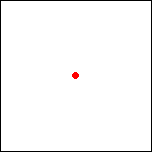lasts .5 rounds n=2lasts 1 round n=3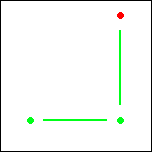lasts 1.5 rounds n=4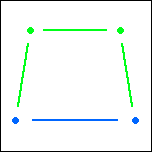lasts 2 rounds
 n=5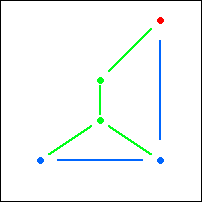lasts 2.5 rounds n=6lasts 3 rounds n=7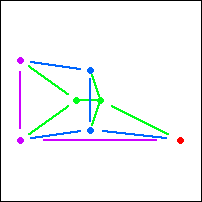lasts 3.5 rounds
 n=8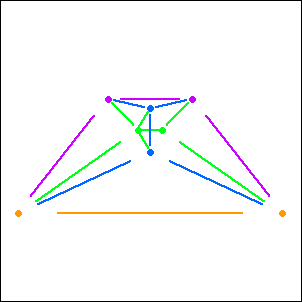lasts 4 rounds n=12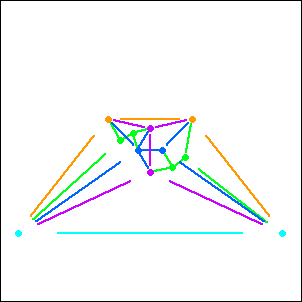lasts 5 rounds
 n=10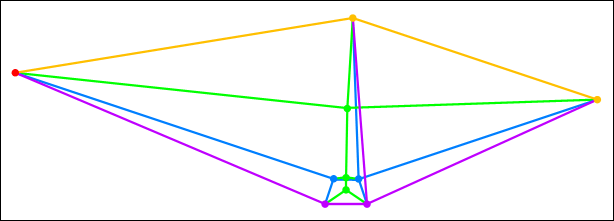(Gavin Theobald)lasts 4.5 rounds

Problem #2:

Joe DeVincentis noted that for small n, n points in a regular n-gon givesn/2distances. He also noted that for some n, circular collections of points from the triangular lattice do better.

Largest Known Number of Points That Determine Only n Distances

 n=0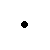1 point n=1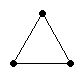3 points n=2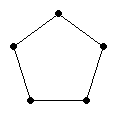5 points n=3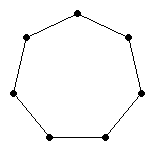7 points n=4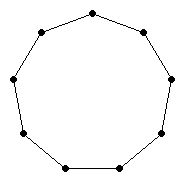9 points
 n=5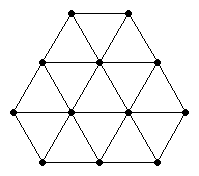12 points n=6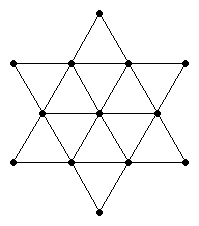13 points n=7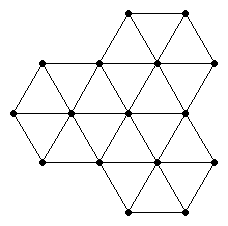16 points
 n=8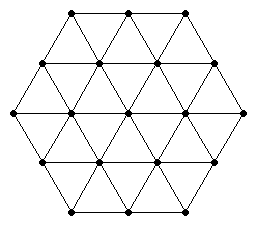19 points n=9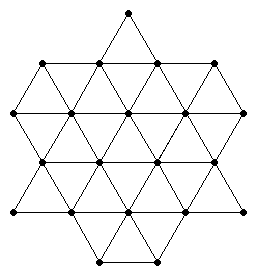21 points

Problem #3:

Joe DeVincentis noted that hiddenness is reflective, so the total number points hidden from each point is even, so when k is odd, n must be even. He also found amazingly small k-hidden arrangements for k≥3.

Corey Plover was able to find all the 1 hidden arrangements, and 2 hidden arrangements with 3k or 4k points.

Minimal k-Hidden Arrangements

 k=1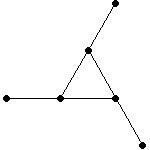n=6 k=2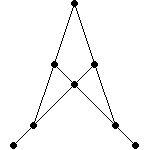n=8 k≥3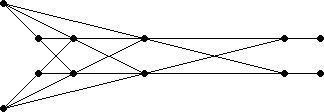(Joe DeVincentis)n=2k+6
Other k-Hidden Arrangements

 k=3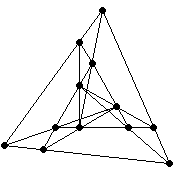n=12 k=4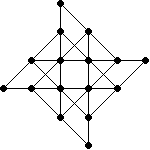(Joe DeVincentis)n=16 k=4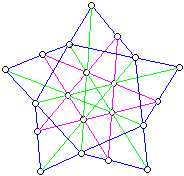(Aron Fay)n=20 k=4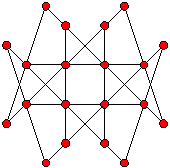n=20 k=5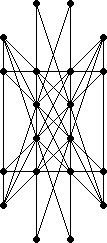(Joe DeVincentis)n=20
Other k-Hidden Arrangements

 k=6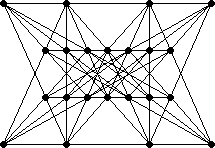(Joe DeVincentis)n=22 k=6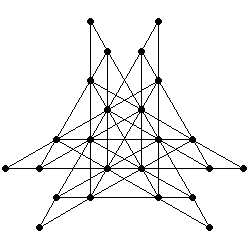(Joe DeVincentis)n=24 k=7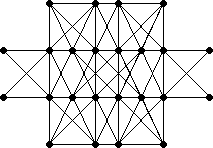(Joe DeVincentis)n=24
 k=8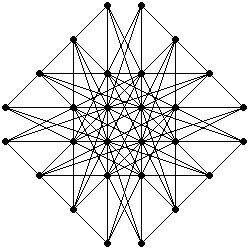(Joe DeVincentis)n=28 k=9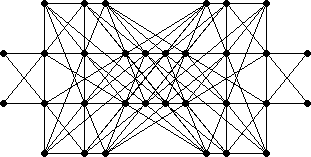(Joe DeVincentis)n=32 k=10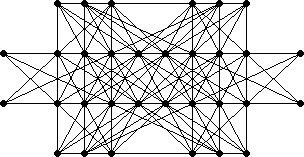(Joe DeVincentis)n=32
 k=11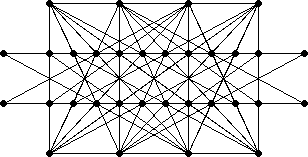(Joe DeVincentis)n=32 k=12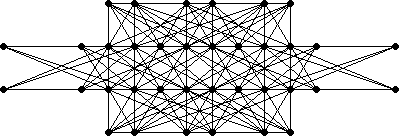(Joe DeVincentis)n=36

Problem #4:

Joe DeVincentis gave several results equalling or bettering the best known graphs.

Fred Helenius found the best known equal 1/4 and 1/5 matchstick graphs.

Gavin Theobald found the best known equal 2/5 matchstick graph.

Jeremy Galvagni and Joe DeVincentis completely solved the problem for m=2, by adding triangles and then skinny diamonds to the small configurations below.

Smallest Known m/n Matchstick Graphs
m/n2345678
1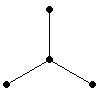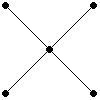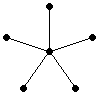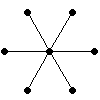2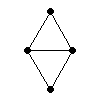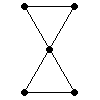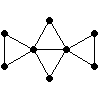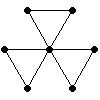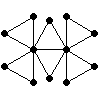3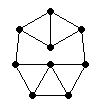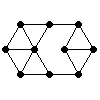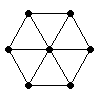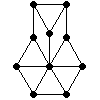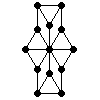m/n56
4(Mike Winkler, 115 matches)(Mike Winkler, 117 matches)

m/n78
4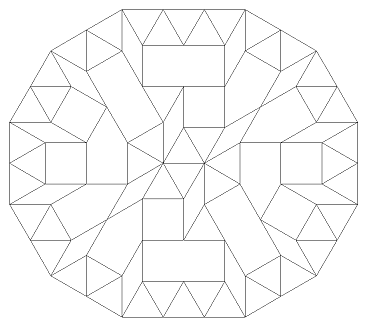(Mike Winkler, 159 matches)(Peter Dinkelacker, 126 matches)

m/n910
4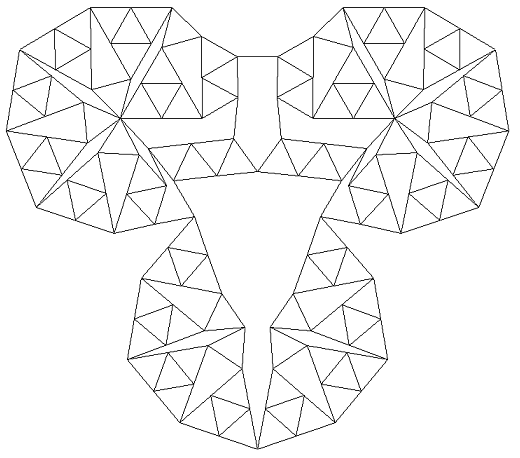(Peter Dinkelacker, 273 matches)(Peter Dinkelacker, 231 matches)

m/n11
4(Mike Winkler, Stefan Vogel, and Peter Dinkelacker, 771 matches)

Minimal Equal m/n Matchstick Graphs
m/n23456
1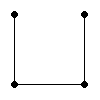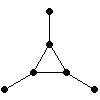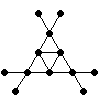(Fred Helenius)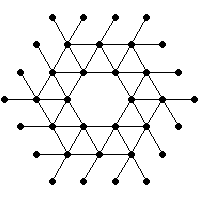(Fred Helenius)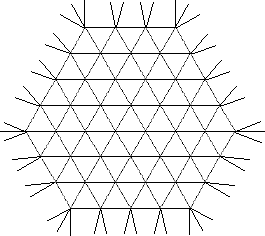(Joe DeVincentis)

2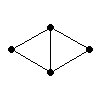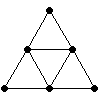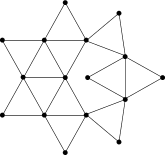(Gavin Theobald)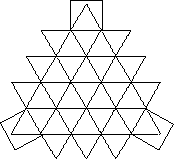(Joe DeVincentis)

3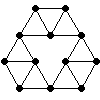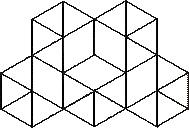(Joe DeVincentis)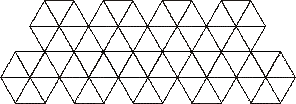(Joe DeVincentis)

m/n56
4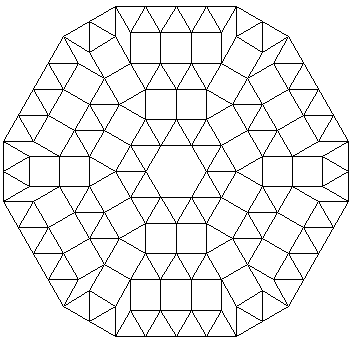(Joe DeVincentis)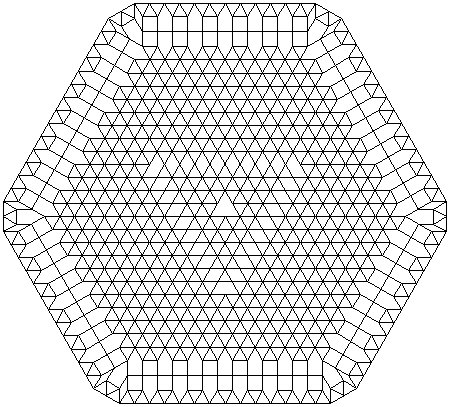(Joe DeVincentis)

Joe DeVincentis suggested at looking at infinite equal matchstick graphs when no finite graph sufficed.

Infinite Equal n Matchstick Graphs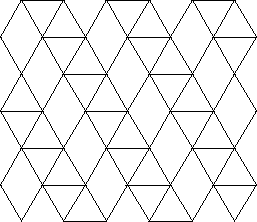n=5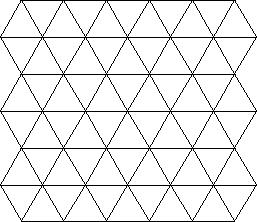n=6

Infinite Equal m/n Matchstick Graphs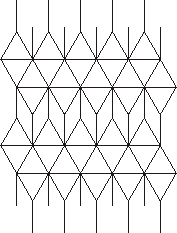m=3 n=7(Joe DeVincentis)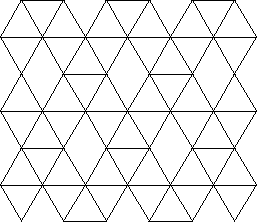m=5 n=6

Joe DeVincentis also suggested at looking at matchstick graphs in three dimensions.

Minimal m/n Matchstick Graphs in Three Dimensions
m/n3456
3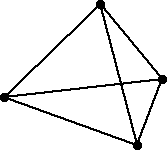(Joe DeVincentis)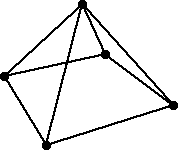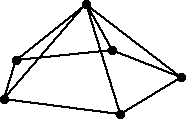4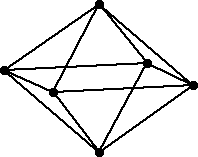(Joe DeVincentis)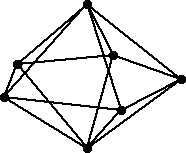(Gavin Theobald)

5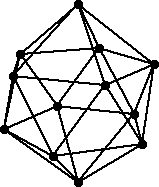(Joe DeVincentis)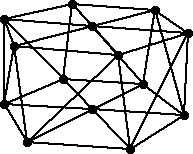I wondered whether there was a 3-regular matchstick graph with no triangles. I found one, but then Gavin Theobald found a smaller one, shown below left. In 2006, Giuseppe Mazzuoccolo found an even smaller one, shown below middle. Then Giuseppe Mazzuoccolo and Sascha Kurz found an even smaller on, shown below right. Is this the smallest one?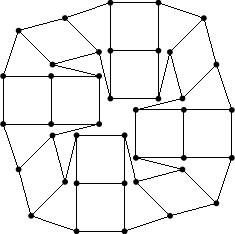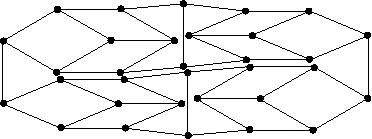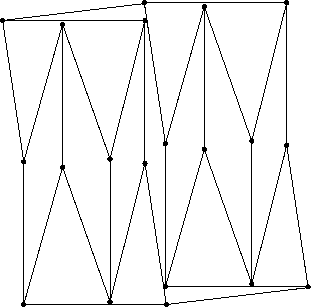They also found a 3-regular matchstick graph with girth 5, shown below. These last two graphs were published in Geombinatorics, Vol XIX (4) in 2009.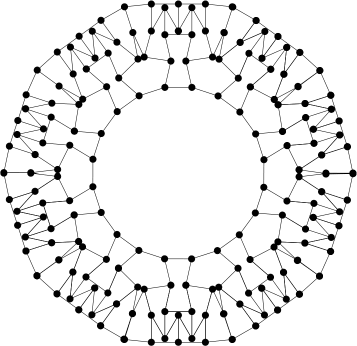Sascha Kurz proved that there are no 5-regular matchstick graphs. His proof is here.

If you can extend any of these results, please e-mail me. Click here to go back to Math Magic. Last updated 3/15/16.